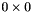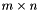linbox
TriangularBlasMatrix< _Field, _Storage > Class Template Reference

Triangular BLAS matrix. More...

#include <blas-matrix.h>Inheritance diagram for TriangularBlasMatrix< _Field, _Storage >:Collaboration diagram for TriangularBlasMatrix< _Field, _Storage >:

## Public Types

typedef Field::Element Element
Element type.

typedef Rep::pointer pointer
pointer type to elements

typedef const pointer const_pointer
const pointer type

typedef const BlasMatrix< Field, RepconstSelf_t
Self typeype.

typedef BlasSubmatrix< Self_tsubMatrixType
Submatrix type.

typedef BlasSubmatrix< constSelf_tconstSubMatrixType
Submatrix type.

typedef Self_t matrixType
matrix type

typedef constSelf_t constMatrixType
matrix type

typedef Self_t blasType
blas matrix type

## Public Member Functions

TriangularBlasMatrix (const Field &F, const size_t m, const size_t n, Tag::Shape x=Tag::Shape::Upper, Tag::Diag y=Tag::Diag::NonUnit)
Constructor for a new TriangularBlasMatrix. More...

TriangularBlasMatrix (const BlasMatrix< Field, Rep > &A, Tag::Shape x=Tag::Shape::Upper, Tag::Diag y=Tag::Diag::NonUnit)
Constructor from a BlasMatrix (copy). More...

TriangularBlasMatrix (BlasMatrix< Field, Rep > &A, Tag::Shape x=Tag::Shape::Upper, Tag::Diag y=Tag::Diag::NonUnit)
Constructor from a BlasMatrix (no copy). More...

TriangularBlasMatrix (const TriangularBlasMatrix< Field, Rep > &A)
Constructor from a TriangularBlasMatrix (copy). More...

template<class Matrix >
TriangularBlasMatrix (const Matrix &A, Tag::Shape x=Tag::Shape::Upper, Tag::Diag y=Tag::Diag::NonUnit)
Generic constructor from a Matrix (no copy). More...

Tag::Shape getUpLo () const
get the shape of the matrix (upper or lower)

Tag::Diag getDiag () const
Is the diagonal implicitly unit ?

void init (const _Field &F, const size_t &r=0, const size_t &c=0)
Allocates a new barematrix (unshaped, unready). More...

template<class Matrix >
BlasMatrixcopy (const Matrix &B)
Make this a (deep)copy of B. More...

size_t rowdim () const
Get the number of rows in the matrix. More...

size_t coldim () const
Get the number of columns in the matrix. More...

size_t getStride () const
Get the stride of the matrix.

size_t & getWriteStride ()
Get a reference to the stride of the matrix. More...

void resize (const size_t &m, const size_t &n, const Element &val=Element())
Resize the matrix to the given dimensions. More...

pointer getPointer ()
.

pointergetWritePointer ()
.

const ElementsetEntry (size_t i, size_t j, const Element &a_ij)
Set the entry at the (i, j) position to a_ij. More...

ElementrefEntry (size_t i, size_t j)
Get a writeable reference to the entry in the (i, j) position. More...

const ElementgetEntry (size_t i, size_t j) const
Get a read-only reference to the entry in the (i, j) position. More...

ElementgetEntry (Element &x, size_t i, size_t j) const
Copy the (i, j) entry into x, and return a reference to x. More...

Self_t transpose (Self_t &tM) const
Creates a transposed matrix of *this. More...

template<bool _IP>
void transpose ()
Transpose (inplace). More...

void reverseRows ()
Reverse the rows of a matrix. More...

void reverseCols ()
Reverse the columns of a matrix. More...

void reverse ()
Reverse the rows/columns of a matrix. More...

template<>
void random (const size_t &b)

Read the matrix from an input stream. More...

std::ostream & write (std::ostream &os) const
Write the matrix in MatrixMarket format.

std::ostream & write (std::ostream &os, Tag::FileFormat f) const
Write the matrix to an output stream. More...

std::ostream & write (std::ostream &os, bool mapleFormat) const

template<class Vector >
VectorcolumnDensity (Vector &v) const
Compute column density. More...

Raw Indexed iterator

Like the raw iterator, the indexed iterator is a method for accessing all entries in the matrix in some unspecified order.

At each position of the the indexed iterator, it also provides the row and column indices of the currently referenced entry. This is provided through it's rowIndex() and colIndex() functions.

IndexedIterator IndexedBegin ()

ConstIndexedIterator IndexedBegin () const

IndexedIterator IndexedEnd ()

ConstIndexedIterator IndexedEnd () const

Row operator[] (size_t i)
Retrieve a reference to a row. More...

ConstRow operator[] (size_t i) const
Retrieve a reference to a row. More...

## Data Fields

pointer _ptr

MatrixDomain< Field > _MD

VectorDomain< Field > _VD

## Protected Attributes

Tag::Shape _uplo
upper or lower triangular

Tag::Diag _diag
unit or non unit diagonal

## Column of rows iterator

The column of rows iterator traverses the rows of the matrix in ascending order.

Dereferencing the iterator yields a row vector in dense format

typedef Subvector< typename Rep::iterator, typename Rep::const_iterator > Row

typedef Subvector< typename Rep::const_iterator > ConstRow

RowIterator rowBegin ()

ConstRowIterator rowBegin () const

RowIterator rowEnd ()

ConstRowIterator rowEnd () const

## Row of columns iterator

The row of columns iterator traverses the columns of the matrix in ascending order.

Dereferencing the iterator yields a column vector in dense format

typedef Subvector< Subiterator< typename Rep::iterator > > Col

typedef Subvector< Subiterator< typename Rep::const_iterator > > ConstCol

typedef Col Column

typedef ConstCol ConstColumn

ColIterator colBegin ()

ConstColIterator colBegin () const

ColIterator colEnd ()

ConstColIterator colEnd () const

## Iterator

The iterator is a method for accessing all entries in the matrix in some unspecified order.

This can be used, e.g. to reduce all matrix entries modulo a prime before passing the matrix into an algorithm.

typedef Rep::iterator Iterator

typedef Rep::const_iterator ConstIterator

Iterator Begin ()

ConstIterator Begin () const

Iterator End ()

ConstIterator End () const

## Detailed Description

### template<class _Field, class _Storage> class LinBox::TriangularBlasMatrix< _Field, _Storage >

Triangular BLAS matrix.

## ◆ TriangularBlasMatrix() [1/5]

 TriangularBlasMatrix ( const Field & F, const size_t m, const size_t n, Tag::Shape x = Tag::Shape::Upper, Tag::Diag y = Tag::Diag::NonUnit )

Constructor for a new TriangularBlasMatrix.

Parameters
 F m rows n cols y (non)unit diagonal x (upp/low)er matrix

## ◆ TriangularBlasMatrix() [2/5]

 TriangularBlasMatrix ( const BlasMatrix< Field, Rep > & A, Tag::Shape x = Tag::Shape::Upper, Tag::Diag y = Tag::Diag::NonUnit )

Constructor from a BlasMatrix (copy).

Parameters
 A matrix y (non)unit diagonal x (upp/low)er matrix

## ◆ TriangularBlasMatrix() [3/5]

 TriangularBlasMatrix ( BlasMatrix< Field, Rep > & A, Tag::Shape x = Tag::Shape::Upper, Tag::Diag y = Tag::Diag::NonUnit )

Constructor from a BlasMatrix (no copy).

Parameters
 A matrix y (non)unit diagonal x (upp/low)er matrix

## ◆ TriangularBlasMatrix() [4/5]

 TriangularBlasMatrix ( const TriangularBlasMatrix< Field, Rep > & A )

Constructor from a TriangularBlasMatrix (copy).

Parameters
 A matrix

## ◆ TriangularBlasMatrix() [5/5]

 TriangularBlasMatrix ( const Matrix & A, Tag::Shape x = Tag::Shape::Upper, Tag::Diag y = Tag::Diag::NonUnit )

Generic constructor from a Matrix (no copy).

Parameters
 A matrix y (non)unit diagonal x (upp/low)er matrix

## ◆ init()

 void init ( const _Field & F, const size_t & r = 0, const size_t & c = 0 )
inherited

Allocates a new barematrix (unshaped, unready).

(Re)allocates a newzero matrix (shaped and ready).

## ◆ copy()

 BlasMatrix& copy ( const Matrix & B )
inlineinherited

Make this a (deep)copy of B.

Assumes same shape. make sure we actually copy

## ◆ rowdim()

 size_t rowdim ( void ) const
inherited

Get the number of rows in the matrix.

Returns
Number of rows in matrix

## ◆ coldim()

 size_t coldim ( void ) const
inherited

Get the number of columns in the matrix.

Returns
Number of columns in matrix

## ◆ getWriteStride()

 size_t & getWriteStride ( )
inherited

Get a reference to the stride of the matrix.

Modify stride this way.

## ◆ resize()

 void resize ( const size_t & m, const size_t & n, const Element & val = Element() )
inherited

Resize the matrix to the given dimensions.

The state of the matrix's entries after a call to this method is undefined

Parameters
 m Number of rows n Number of columns val

## ◆ setEntry()

 const _Field::Element & setEntry ( size_t i, size_t j, const Element & a_ij )
inherited

Set the entry at the (i, j) position to a_ij.

Parameters
 i Row number, 0...rowdim () - 1 j Column number 0...coldim () - 1 a_ij Element to set

## ◆ refEntry()

 _Field::Element & refEntry ( size_t i, size_t j )
inherited

Get a writeable reference to the entry in the (i, j) position.

Parameters
 i Row index of entry j Column index of entry
Returns
Reference to matrix entry

## ◆ getEntry() [1/2]

 const _Field::Element & getEntry ( size_t i, size_t j ) const
inherited

Get a read-only reference to the entry in the (i, j) position.

Parameters
 i Row index j Column index
Returns
Const reference to matrix entry

## ◆ getEntry() [2/2]

 _Field::Element & getEntry ( Element & x, size_t i, size_t j ) const
inherited

Copy the (i, j) entry into x, and return a reference to x.

This form is more in the Linbox style and is provided for interface compatibility with other parts of the library

Parameters
 x Element in which to store result i Row index j Column index
Returns
Reference to x

## ◆ transpose() [1/2]

 Self_t transpose ( Self_t & tM ) const
inherited

Creates a transposed matrix of *this.

Parameters
 [in] tM
Returns
the transposed matrix of this.

## ◆ transpose() [2/2]

 void transpose ( )
inherited

Transpose (inplace).

If rows and columns agree, we can transpose inplace.

## ◆ reverseRows()

 void reverseRows ( )
inherited

Reverse the rows of a matrix.

This is done inplace. Let J=antiDiag(1) (or the matrix of the reverse permutation or the matrix (i,j) = (i+j+1==m)). Then, we compute A <- J.A;

## ◆ reverseCols()

 void reverseCols ( )
inherited

Reverse the columns of a matrix.

This is done inplace. This is A <- J.A

## ◆ reverse()

 void reverse ( )
inherited

Reverse the rows/columns of a matrix.

This is done inplace. This is A <- J.A.J

## ◆ random()

 void random< size_t > ( const size_t & b )
inherited
Bug:
does not work for submatrices.
Todo:
b should be the random generator

 std::istream & read ( std::istream & file )
inherited

Read the matrix from an input stream.

The stream is in SMS, DENSE, or MatrixMarket format and is autodetected.

Parameters
 file Input stream from which to read

## ◆ write() [1/2]

 std::ostream& write ( std::ostream & os, Tag::FileFormat f ) const
inlineinherited

Write the matrix to an output stream.

Parameters
 os Output stream to which to write f write in some format (Tag::FileFormat::Format). Default is Maple's.

## ◆ write() [2/2]

 std::ostream& write ( std::ostream & os, bool mapleFormat ) const
inlineinherited
Deprecated:
Only for compatiblity.

## ◆ operator[]() [1/2]

 BlasMatrix< _Field, _Rep >::Row operator[] ( size_t i )
inherited

Retrieve a reference to a row.

Since rows may also be indexed, this allows A[i][j] notation to be used.

Parameters
 i Row index
Bug:
Rows and Cols should be BlasVectors

## ◆ operator[]() [2/2]

 BlasMatrix< _Field, _Rep >::ConstRow operator[] ( size_t i ) const
inherited

Retrieve a reference to a row.

Since rows may also be indexed, this allows A[i][j] notation to be used.

Parameters
 i Row index
Bug:
Rows and Cols should be BlasVectors

## ◆ columnDensity()

 Vector & columnDensity ( Vector & v ) const
inherited

Compute column density.

Parameters
 v

## ◆ _ptr

 pointer _ptr
inherited
Bug:
why public ?

## ◆ _MD

 MatrixDomain _MD
inherited
Bug:
why public ?

## ◆ _VD

 VectorDomain _VD
inherited
Bug:
why public ?

The documentation for this class was generated from the following files: